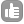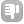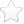0Hi guys, I'm stuck in attempting this question and need some help understanding how to answer it. Q.) The chemical shift of H in benzene is 7.4 ppm. what will the resonance frequency be at 200mhz I know that the the resonance frequency is proportional to the magnetic field intensity and think I need to use the Larmor equation, Vo = gamma Bo/2pi , but can't quite understand how? Please help. Thank-you. asked Feb 12 '14 at 11:541The Larmor equation that you give neglects to include the screening constant, otherwise known as the chemical shift. This is what your 7.4 ppm represents. When you say a "200 MHz" spectrometer, that means that protons resonante somewhere around 200 Mhz, the exact frequency of which depends on the exact magnetic field, including any B0 or Z0 offset, and the chemical shift of the substance that you are observing. Vo = gamma Bo(1-sigma)/2pi Where sigma is essentially the chemical shift, although referenced to gamma for the bare nucleus in this case. Since bare nuclei are hard to come by, we use a scale referenced to a standard, usually TMS for protons (which I think is about 17 ppm to low frequency of the bare proton). On a 200 MHz spectrometer from one particular vendor (Bruker), the field will typically be adjusted so that the protons of TMS resonate at exactly 200.13 MHz. This means that benzene will be 7.4 ppm or 1481 Hz higher (I know that seems strange, but that is just the way chemical scale is defined) in frequency, or 200.131481 MHz. Chemical shift is a relative dimensionless parameter and as such only defines an absolute frequency when refered to the frequency of a standard. answered Feb 13 '14 at 12:23Kirk Marat 7110ppm = (frequency:sample - frequency:reference)/(operating frequency of spectrometer) Reference ppm is usually 0ppm so frequency:reference = operating frequency of spectrometer. delta frequency = ppm * operating freq (This gives you distance from resonant frequency) absolute frequency = operating frequency + delta frequency You can use the exact resonant frequency of your spectrometer for more accuracy. answered Feb 13 '14 at 07:31It is important to keep the 1/1,000,000 factor of the "ppm" units when determining the delta frequency. Without it, the delta frequency calculation results in many MHz of error. - Jerry Hirschinger (Feb 20 '14 at 10:55)0Maybe it's better if you ask the question like this: The chemical shift of H in benzene is 7.4 ppm. what will the resonance frequency be relative to reference TMS, at 200MHz? The answer is 7.4 * 200 in unit Hz, which is the relative resonance frequency. If you want to get the absolute resonance frequency at 7.4 ppm or any other position, the key is to get the absolute frequency right for the reference TMS. You need the basic frequency parameter from the type of NMR instrument you are referring to and the chemical shift referencing method for your instrument. Dejian seeking new job opportunities in NMR (dejian "at" gmail "dot" com) answered Feb 16 '14 at 09:03dejian 11 It is important to keep the 1/1,000,000 factor of the "ppm" units when determining the delta frequency. Without it, the delta frequency calculation results in many MHz of error. - Jerry Hirschinger (Feb 20 '14 at 10:56)0Try to know the two terms "Spectrometer Frequency" and "Resonance Frequency" By way of trying to get to know the difference, then you may arrive at a solution for the problem posed as a question here in these postings. If still there is ambiguities, clearly spell out the ambiguity again in these boxes. S.Aravamudhan answered Feb 17 '14 at 23:340"When you say a "200 MHz" spectrometer, that "means that protons resonante somewhere around 200 Mhz", for an unambiguous calculation, Try to calculate the Resonance frequency for the Reference Compund the "TMS", this requires the frequency of the nucleus based on its gamma value (bare nucleus), add the shielding value for the reference and calculate for the experimental measured field/ or calibrate with the set Transmitter frequency of the Spectrometer. answered Feb 28 '14 at 01:0007.0460. Tesla for 300 MHz (protons) 4.6973333 Tesla for 200 MHz If the Magnetic Field is 4.6973333 Tesla, then bare proton resonance frequency is 200 MHz. Then, if you have a TMS sample, in the Spectrometer with 4.6973333 Tesla magnetic field, then according to the standard listed values, with reference to bare nucleus resonates at 30 ppm lower field (higher frequency) direction to TMS. In other words, at 4.6973333 Tesla, TMS resonates at 30-ppm lower frequency to bare Nucleus resonance frequency of 200 MHz. 30 ppm corresponds to 6000 Hz (6 KHz) frequency at 4.6973333 Tesla Magnetic field. Thus, at 4.6973333 Tesla, TMS would resonate at (200,000000-6000=199994000 Hz) 199.994 MHz. Since benzene protons resonate 7.4 ppm lowfield (high frequency) side of TMS, at 4.6973333 Tesla benzene proton resonance frequency would be 199.994 MHz + (7.4 x 200) Hz =199.994 MHz + 1,480 Hz =199.994 MHz + 1.480. KHz =199.994 MHz + 0.001480 MHz =199.995480 MHz answered Apr 02 '14 at 00:25toggle preview

Tags:

×26
×8

Asked: Feb 12 '14 at 11:54

Seen: 20,545 times

Last updated: Apr 02 '14 at 00:25